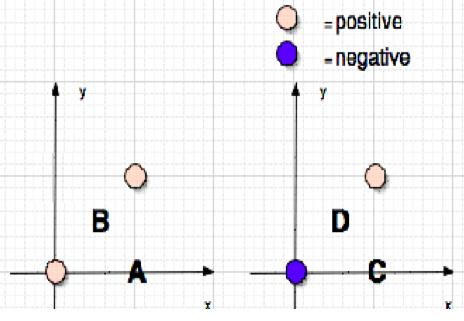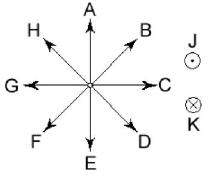Teaching Physics with the Physics Suite Edward F. Redish Problems Sorted by Type | Problems Sorted by Subject | Problems Sorted by Chapter in UP

Electric Forces

 In the figure at the right are shown two settings of positive and negative charges. The setting on the left has two positive charges; the setting on the right has one negative charge and one positive charge. Each of the charges has the same magnitude. (i) In each setting, two positions are labeled. If a test charge were to measure the field at each position, rank the magnitude of the electric field the charge would measure at the four places, indicating if any are 0. (Like this E=F>G>H=0) (ii) If a test charge of magnitude twice as large as the original test charge were placed at point A, how would the force it feels compare to the force felt by the original test charge when it was placed at point A?1. same
2. twice as big
3. half as big
4. other

iii) How would the electric field measured at point A by the test charge in ii) compare to the electric field measured at point A by the test charge in i)?

1. same
2. twice as big
3. half as big
4. other
 iv) For the test charge in i) placed at point C, indicate which of the directions in the compass rose at the left best represents the direction of the electric force felt by the charge. (J points out of the page, K points into the page.)